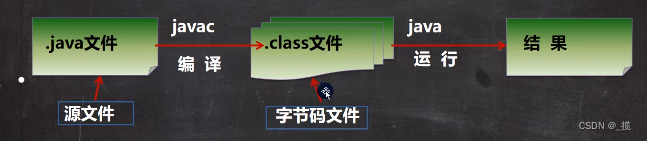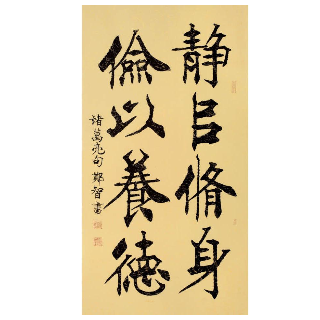# Java初级笔记-第二章

+关注继续查看

2.1 标识符

A-Z、a-z、0-9、_、\$

 标识符不可以是中文。
2.2 标识符的使用规范

 包名全小写。Eg:axx.bxxx.cxx
 类名单词的首字母大写（大驼峰规则）。Eg:AxxDxxGxx
 变量名、方法名:第二个单词才开始首字母大写（小驼峰规则）。Eg:axxBxxCxx
 常量名：全大写，单词之间以_作为分隔。
2.3 分隔符
( ) { } [] “ ” ‘ ‘, . ; 等符号作为Java源代码中的分隔符使用，分隔符是将程序代码进行分析的依据，Javac根据分隔符进行分析Java源程序。
() 与方法的声明、调用有关成对出现，一般出现在方法定义与使用的地方。括号的另一作用是优先进行。
{} 与变量的作用域、语句功能分块有关，成对出现。
[] 与数组有关，成对出现。
“ ” 一般用作划分字符串，例如在“xxx”中xxx就是一个字符串。
‘ ‘ 表示中间内容是字符，
. 类与变量的分隔符，说明.后是.前类的一个变量（常量）。
; 用于流程图中处理框或输入输出框的后，用;表示一条命令（表达）结束。单个的;也代表空语句。
, 用于分隔变量，语句等。

2.3 关键字（保留字）

2.3.1 关键字

abstract    break   byte    boolean catch   case    class   char    continue default    double  do  else    extends false   final   float   for finally if  import  implements  int interface   instanceof  long    length native   new null    package private protected   public  return  switch short    static  super   try true    this    throw   throws  void threadsafe transient   while   synchronized

2.3.2 关键字解释

1 abstract 抽象方法，抽象类的修饰符
2 assert 断言条件是否满足
3 boolean 布尔数据类型
4 break 跳出循环或者label代码段
5 byte 8-bit 有符号数据类型
6 case switch语句的一个条件
7 catch 和try搭配扑捉异常信息
8 char 16-bit Unicode字符数据类型
9 class 定义类
0 const 未使用
1 continue 不执行循环体剩余部分
2 default switch语句中的默认分支
3 do 循环语句，循环体至少会执行一次
4 double 64-bit双精度浮点数
5 else if条件不成立时执行的分支
6 enum 枚举类型
7 extends 表示一个类是另一个类的子类
8 final 表示一个值在初始化之后就不能再改变了

9 finally 为了完成执行的代码而设计的，主要是为了程序的健壮性和完整性，无论有没有异常发生都执行代码。
0 float 32-bit单精度浮点数
1 for for循环语句
2 goto 未使用
3 if 条件语句
4 implements 表示一个类实现了接口
5 import 导入类
6 instanceof 测试一个对象是否是某个类的实例
7 int 32位整型数
8 interface 接口，一种抽象的类型，仅有方法和常量的定义
9 long 64位整型数
0 native 表示方法用非java代码实现
1 new 分配新的类实例
2 package 一系列相关类组成一个包
3 private 表示私有字段，或者方法等，只能从类内部访问
4 protected 表示字段只能通过类或者其子类访问

5 public 表示共有属性或者方法
6 return 方法返回值
7 short 16位数字
8 static 表示在类级别定义，所有实例共享的
9 strictfp 浮点数比较使用严格的规则
0 super 表示基类
1 switch 选择语句
2 synchronized 表示同一时间只能由一个线程访问的代码块
3 this 表示调用当前实例

4 throw 抛出异常
5 throws 定义方法可能抛出的异常
6 transient 修饰不要序列化的字段
7 try 表示代码块要做异常处理或者和finally配合表示是否抛出异常都执行finally中的代码
8 void 标记方法不返回任何值
9 volatile 标记字段可能会被多个线程同时访问，而不做同步
0 while while循环
2.4 Java的数据类型

2.4.1 常量

1) 标识常量

final 数据类型 常量名=value[,常量名=value …];
final double PI=3.14,PI2=3.1415926,PI3=1.0000000000;// 常量命名规则一般使用大写字母
2）直接常量（字面常量）

21，45，789，1254，-254 表示整型量；
12L，123l， -145321L 尾部加大写字母L或小写字母l表示该量是长整型量；（long）
456.12，-2546，987.235 表示双精度浮点型量，也可以加D或d；
4567.2145F，54678.2f 尾部加大写字母F或小写字母f表示单精度浮点型量。(float)
 在使用定义语法时，中括号[]中的内容代表可有可无。
2.4.2 变量

2.4.3 变量的作用域
{} 规定了变量的有效区域——作用域；

/**

*/
import java.util.*;

public class HelloWorld {

static int intVar1 = 10;

public void display(){
int intVar2 = 200;
intVar1 = intVar1 + intVar2;
System.out.println("intVar1=" + intVar1);
System.out.println("intVar2=" + intVar2);

{
intVar2 = 10;
int intVar3 = 10;
}

{
intVar3 = 0; //出错了，不可以访问与自己同级的{}
}
}

public static void main(String [] args){
int intVar3 = intVar1 * 2;
//int intVar2 = 10;
System.out.println("intVar1=" + intVar1);
//System.out.println("intVar2=" + intVar2);
System.out.println("intVar3=" + intVar3);
System.out.println("我爱学Java");
}
}

2.4.4 基本数据类型
Java的基本数据类型分为整型（byte,short,int,long）、字符型(char)、布尔型(boolean)、浮点型(double,float)

byte 8 1 -27～27-1
short 16 2 -215～215-1
int 32 4 -231～231-1
long 64 8 -263～263-1
char 16 2 任意字符
boolean 8 1 true , false
float 32 4 -3.4E38(3.4×1038)～3.4E38(3.4×1038)
double 64 8 -1.7E308(1.7×10308)～1.7E308(1.7×10308)
2.4.5 整型

 数字的进制
HEX BIN OCT DEC HEX BIN OCT DEC
0x0 0000 00 0 0x8 1000 010 8
0x1 0001 01 1 0x9 1001 011 9
0x2 0010 02 2 0xA 1010 012 10
0x3 0011 03 3 0xB 1011 013 11
0x4 0100 04 4 0xC 1100 014 12
0x5 0101 05 5 0xD 1101 015 13
0x6 0110 06 6 0xE 1110 016 14
0x7 0111 07 7 0xF 1111 017 15
020

boolean Boolean int Integer
byte    Byte    long    Long
char    Character   float   Float
short   Short   double  Double
/**

*/
import java.util.*;

public class HelloWorld {
public static void main(String [] args) {
//byte

int intVar = 98303;// 所有的变量都需要先定义后使用

System.out.println("intVar的值：" + intVar);// 输出intVar的值
System.out.println("intVar的二进制：" + Integer.toBinaryString(intVar)); // intVar的二进制
System.out.println("intVar的十六进制：" + Integer.toHexString(intVar));// intVar 十六进制
System.out.println("int 型数据的最大值：" + Integer.MAX_VALUE);
System.out.println("int 型数据的最小值：" + Integer.MIN_VALUE);//封装类中的

short shortVar = (short)0x8000; // 使用空间从大到小赋值时，要进行强制类型转换
// (基本数据类型) 常值 是强制转换的格式语法
System.out.println("shortVar的值：" + shortVar);// 输出intVar的值
System.out.println("shortVar的二进制：" + Integer.toBinaryString(shortVar)); // intVar的二进制
System.out.println("shortVar的十六进制：" + Integer.toHexString(shortVar));// intVar 十六进制

//long
long longVar = 90303;// 使用空间从小向大的里放值时，不用强制转换

longVar = -1; // 占用4个字节

longVar = 0xFFFFFFFFFFFFFFFFL;
// L 或l 表示这个常值是长整型的，要占用8个字节的空间
System.out.println("我爱学Java");
}
}

2.4.6 字符型
char 占用2个字节
char ch = ‘a’;
char ch = ‘中’
java 中char中的数值是十六进制的UNICODE，可以包含任意的语言字符。

/**

*/
import java.util.*;

public class HelloWorld {
public static void main(String [] args) {
char ch = 'a';
ch = '中';
int intCh = ch;
ch = '\u3012';
System.out.println(Integer.toHexString(intCh));
System.out.println(ch);
}
}

\b 退格键 backspace
\t 制表格式 tab
\n 换行符号
\f 进纸、分页
\r 回车键 enter
\ 反斜杠
\’ 单引号
\” 双引号

2.4.7 浮点型

/**

*/
import java.util.*;

public class HelloWorld {
public static void main(String [] args) {
//float floatVar = 1; // 可以 自动 进行 类型转换
//float floatVar = 1.0;// 不可以自动 进行类型转换。
float floatVar = 1.0F;// F 表示的是float类型的常值。
double doubleVar = 1.0; // Java 缺省的浮点类型是double
floatVar = (float)doubleVar;
}
}
2.4.8 布尔型
boolean 只有两种值 true false;占用1BYTE
/**

*/
import java.util.*;

public class HelloWorld {
public static void main(String [] args) {
boolean boolVar1 = false;
boolean boolVar2 = true;
System.out.println( boolVar1);
System.out.println( boolVar2);
}
}

2.5运算符和表达式

 表达式是由操作数（变量或常量）和运算符按一定的语法形式组成的符号序列。
 一个常量或一个变量名是最简单的表达式。
 表达式是可以计算值的运算式，一个表达式有确定类型的值。

1 分隔符 [] () ; ,
2 单目运算、字符串运算 + -（正数负数） ++ – ~ ! (类型转换符) *右到左
3 算术乘除运算 * / % 左到右
4 算术加减运算 + - 左到右
5 移位运算 << >> >>> 左到右
6 大小关系运算、类运算 < > <= >= instanceof 左到右
7 相等关系运算 == != 左到右
8 按位与，非简洁与 & 左到右
9 按位异或运算 ^ 左到右
10 按位或，非简洁或 | 左到右
11 简洁与 && 左到右
12 简洁或 || 左到右
13 三目条件运算 ?: *右到左
14 简单、复杂赋值运算 = = /= %= += -= <<= >>= >>>= &= ^= |= 右到左

2.5.1 赋值运算符

= 通知CPU把一个数放到变量名所代表的内存中。

/*

byte->short,char->int->long->float->double
*/
import java.util.*;
public class HelloWorld1 {
public static void main(String [] aaa)
{
int a;
float b;
long d=0L;
a = 0;
b = d;
d = (long)b;
float c = 0.5f;
b = 1.5F+c;
a = (int)b;
System.out.println(a);
}
}

Java在进行不同类型之间的数据进行赋值的时候，会自动处理类型转换，类型转换标准是：

byte  short , char  int  long  float  double

2.5.2 算数运算符

/*

*/
import java.util.*;
public class HelloWorld1 {
public static void main(String [] args)
{
//int a,b = 0;// 对于b来说，=0叫初始化
int a = 0 ,b = 0,c = 0;
int d; //定义一个变量，整数类型，用d表示。

double aaaa = 0.0;

a = 0;// 只是纯粹的赋值。
// Java中的规定是变量在使用（读取）之前一定要赋值。
// 我们的规定，定义了变量就要初始化。
a = 3;
b = 2;
c = a + b;

System.out.println(a + "+" + b + "=" + c);
c ++;// c = c + 1;
System.out.println("c++=" + c);
c --;// c = c - 1;
System.out.println("c--=" + c);
c = ++a;// a = a+1; c = a;
System.out.println("c = ++ a的值是：" + c);
a = 3;
c = a ++;// c = a; a = a+1;
System.out.println("c = a ++的值是：" + c);
System.out.println(a);
// 没有** // 并且除数不可为0

// % 叫模运算 取余数的意思。
a = 100;
b = 0;
c = a%b;
System.out.println(a + "%" + b + "=" + c);

c = a/b;
System.out.println(a + "/" + b + "=" + c);

}
}

++ 和 – 有先运算或后运算之说， 前（后）++ 或 前（后） –，前++（–）要先计算后赋值，后++(–)要先赋值后计算。

+=,-=,*= ,/=, %=

/*

+=,-=,*= ,/=, %=
*/
import java.util.*;
public class HelloWorld1 {
public static void main(String [] args)
{
//int a,b = 0;// 对于b来说，=0叫初始化
int a = 100 ,b = 2,c = 3;
int d; //定义一个变量，整数类型，用d表示。
System.out.println("b=" + b);

b += 100;// b = b + 100;
b -= 100;// b = b - 100;
b *= 100;// b = b * 100;
b /= 100;// b = b / 100;
b %= c;  // b = b % c;
System.out.println("b += 100后 b=" + b);
}
}

2.5.3 关系运算符

/*

> 、< 、 >= 、<= 、==、!=
*/
import java.util.*;
public class HelloWorld1 {
public static void main(String [] args)
{
//int a,b = 0;// 对于b来说，=0叫初始化
int a = 100 ,b = 2,c = 3;

boolean d = false; //定义一个变量，整数类型，用d表示。初始值是  0
d = c == b ++;

System.out.println("d=" + d);
}
}

2.5.4 逻辑（布尔）运算符

！逻辑非(否) && 逻辑与（并且） || 逻辑或（或者）

!true = false
!false = true

true && true = true
true && false = false
false && false = false
false && true = false

true || true = true
true || false = true
false || true = true
false || false = false
2.5.5 位运算符

/*

、 <*/
import java.util.*;
public class HelloWorld1 {
public static void main(String [] args)
{
//int a,b = 0;// 对于b来说，=0叫初始化
// 对于右移来说是 /2 操作，符号位保持不变(补符号位)。
// 对于左移来说是 *2 操作，符号位舍了，右边补0
// 对于int 型的数据来说 移位操作应该在1-31范围内，否则系统会对移位长度进行模运算。
// 比如说int i;i<<=35 最终结果可为 i<<=3;因为 35%32=3
// >>> 所的有位都向右移动，高位补0（不带符号的右移）
// 不带符号的意思是把所有移动单元看成一个整体，没有符号位了

int a = 100;
System.out.println(a+”>>1 = ” + (a>>1));
System.out.println(a+”>>2 = ” + (a>>2));

a = 1;
System.out.println(a+”<<32 = ” + (a<<32)); //a
System.out.println(a+”<<31 = ” + (a<<31) + ” ” + Integer.toHexString(a<<31)); //a

a = -1;
// 0x7fff >>1 -> 0x3fff
// 0x8000 >>1 -> 0xc000

System.out.println( Integer.toHexString(0x80000000 >>1));
System.out.println( Integer.toHexString(0x7FFFFFFF >>1));

System.out.println(a+”>>1 = ” + (a>>1));
System.out.println(a+”>>31 = ” + (a>>31));

// 0x80000000 >> 1 ->0x40000000
System.out.println( Integer.toHexString(0x80000000 >>>32));
}
}容:2的/*

、 << 、*/
import java.util.*;
public class HelloWorld1 {
public static void main(String [] args) {
for(int i=0;i<32;i++) {
System.out.println(“1<<” + i + “=” + (1<

/*

>> 、 << 、>>>
*/
import java.util.*;
public class HelloWorld1 {
public static void main(String [] args)
{
int a = 0x00000010;
int b = 0xFFFFFFFF;
// & : 0 & 1 = 0 ;1 & 1 = 1; 1 & 0 = 0;
System.out.println(Integer.toHexString(b & a));// 0x00000010

b = 0x7FFFFFFF;
a = 0x80000000;
// |: 1 | 0 = 1; 1 | 1 = 1; 0 | 0 = 0  1 | 0 = 1
System.out.println(Integer.toHexString(b | a));// 0xFFFFFFFF

b = 0x7FFFFFFF;
a = 0x80000000;
// ^ : 1 ^ 0 = 1; 0 ^ 0 = 0; 1 ^ 1 = 0 ; 0 ^ 1 = 1;
System.out.println(Integer.toHexString(b ^ a));// 0xFFFFFFFF

// ~ : ~1 = 0 ; ~0 = 1;
System.out.println(Integer.toHexString(~a) );// 0x7FFFFFFFF
System.out.println(0^0);// 0
//a = 0x7;  b = 0x70; 0x7= 0x07 // 要进行右对齐运算，空位补0

}
}

&=、|=、^=、<<=、>>=、>>>=

2.5.6 条件运算符(？：)

/*

*/
import java.util.*;
public class HelloWorld1 {
public static void main(String [] args)
{
String str = "";// 字符串变量
String strJG = "及格";
String strBJG = "不及格";
//strJG = "呵呵"; 不想被更改结果，请用 final修饰
// 小明的成绩 cj;
int cj = 70;
//str = cj >= 60 ? "及格" : "不及格";
str = cj >= 60 ? strJG : strBJG;
System.out.println(str);
}
}

2.5.7 字符串连接符+

/*

+ 还可以用于连接字符串
""

*/
import java.util.*;
public class HelloWorld1 {
public static void main(String [] args)
{
int a = 1 ,b = 2;
System.out.println(a + b);
System.out.println("" + a + b);
System.out.println("XXXX：" + (a + b));// 把每一个数字看成了字符串，互相连接。
String strA  = "你",strB="好";
String strC = strA + strB;// 纯粹字符串相加（连接）
System.out.println(strC);// 输出你好
}
}

2.5.8 其它运算符

new 、instanceofJava初级-Spring常见面试题总结
Java初级-Spring常见面试题总结
105 0JAVA初级学习笔记（韩顺平1-263）
JDK 的全称(Java Development Kit Java 开发工具包)
65 067 0Java 初级 面试前准备
Java 初级 面试前准备
65 0Java开发初级6.24.3
5.在Git使用过程中，进行Git配置的操作命令是哪个（） A. config B. config -g C. config -a D. git config 相关知识点： 在git中，经常使用git config 命令用来配置git的配置文件，git配置级别主要有：仓库级别 local 【优先级最高】、用户级别 global【优先级次之】、系统级别 system【优先级最低】 正确答案：D 10.RDBMS是什么？ A. Rela Database Management Systems B. Relational Database Management Systems C. Relation
55 0Java开发初级6.24.2
3.Java网站src/main/java目录保存的是什么资源？ A. Java源代码文件 B. 测试代码 C. JavaScript、CSS等文件 D. 图片资源 正确答案：A 4.什么是索引Index？ A. SQL数据库里的表管理工具 B. SQL数据库里的查询工具 C. SQL数据库里的目录工具 D. SQL数据库用来加速数据查询的特殊的数据结构 正确答案：D
71 0Java开发初级6.24.1
1.下面关于泛型的描述中错误的一项是？ A. “? extends 类”表示设置泛型上限 B. “? super 类”表示设置泛型下限 C. 利用“?”通配符可以接收全部的泛型类型实例，但却不可修改泛型属性内容 D. 如果类在定义时使用了泛型，则在实例化类对象时需要设置相应的泛型类型，否则程序将无法编译通过 相关知识点： https://edu.aliyun.com/course/35 正确答案：D 2.下列选项中属于SVN中控制鉴权用户访问版本库的权限默认权限的是() A. write B. read C. none D. null 相关知识点： auth-access:取值范围为"writ
115 0Java开发初级6.23.3
5.在Git使用过程中，进行Git配置的操作命令是哪个（） A. config B. config -g C. config -a D. git config 相关知识点： 在git中，经常使用git config 命令用来配置git的配置文件，git配置级别主要有：仓库级别 local 【优先级最高】、用户级别 global【优先级次之】、系统级别 system【优先级最低】 正确答案：D 10.RDBMS是什么？ A. Rela Database Management Systems B. Relational Database Management Systems C. Relation
57 0Java开发初级6.23.2
3.Java网站src/main/java目录保存的是什么资源？ A. Java源代码文件 B. 测试代码 C. JavaScript、CSS等文件 D. 图片资源 正确答案：A 4.什么是索引Index？ A. SQL数据库里的表管理工具 B. SQL数据库里的查询工具 C. SQL数据库里的目录工具 D. SQL数据库用来加速数据查询的特殊的数据结构 正确答案：D
118 0Java开发初级6.23.1
1.下面关于泛型的描述中错误的一项是？ A. “? extends 类”表示设置泛型上限 B. “? super 类”表示设置泛型下限 C. 利用“?”通配符可以接收全部的泛型类型实例，但却不可修改泛型属性内容 D. 如果类在定义时使用了泛型，则在实例化类对象时需要设置相应的泛型类型，否则程序将无法编译通过 相关知识点： https://edu.aliyun.com/course/35 正确答案：D 2.下列选项中属于SVN中控制鉴权用户访问版本库的权限默认权限的是() A. write B. read C. none D. null 相关知识点： auth-access:取值范围为"writ
70 0

Java编程入门1493330

JAVA开发手册1.5.0